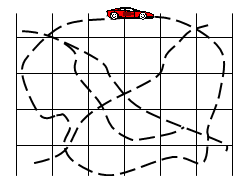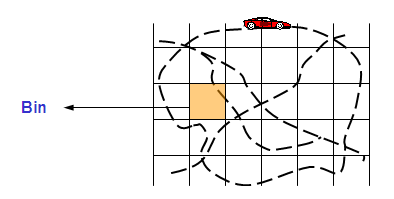## What and Why Drive Test in RF Network

What and Why Drive Test in RF Network, rf signal strength measurements, drive test is performed, drive test, variations due, sampling rate.

INTRODUCTION• Predesign drive test for measurement integration
• This is at beginning of design when no site has been built or even selected. All test sites are temporary.
• Drive test is performed mostly for characterization of propagation and fading effects in the channel. The object is to collect field data to optimize and adjust the prediction model for preliminary simulations.
• Post design drive test for site verification / optimization
• Drive test is performed to verify if they meet the coverage objectives.
• Overlaps are checked for hand-offs.• In field measurement we have to collect variations due to propagation and slow fading.
• The received signals are typically sampled and averaged over spatial windows called bins.
• There are several sampling issues to be considered like
• Sampling rate
• Averaging window
• Number of bins to be measured

SAMPLING CRITEREA

• When measuring the RF signal strength certain sampling criteria must be met to eliminate the short-term fading components from the long-term component ( I.e. log normal fading )
• The RF signal strength measurements must be taken over a radio path or mobile path distance interval of 40l, where l is the wavelength of the RF signal.
• If the distance interval is too short, the short term variation cannot be smoothed out and will affect the local mean.
• If the distance interval is too long, the averaged output cannot represent the local mean since it washes out the detailed signal changes due to the terrain variations.
• The number of RF measurements taken within the 40l distance should be greater than 50.
• Depending on the speed of the vehicle during the drive test, the sampling interval in time is selected.
• Measurements have to be stopped whenever the vehicle is not moving.
• If    f = 1900MHZ, then
• l = 3 * 108 / 1900 * 106
•  =0.158 m
• 40 l = 40 * 0.158
• = 6.32 m
• 50 measurements must be recorded every 6.32m or 1 measurement every 0.1264m
• The conversion from sampling distance to mobile velocity can be done as follows
• minimum sampling rate ( per second ) = v / (0.1264 m/sample)
• If velocity of vehicle is 50 kph then
• Sampling rate( per second )  = (50000/ 3600) / 0.1264

= 110 samples / sec

• TEMS kit cannot be used for this purpose as it can report RF signal strength measurements at a maximum rate of 1 sample per second

WINDOW SIZE

• In field measurements the interest is on local averages of received signals.
• The size of averaging window has to be small enough to capture slow variations due to shadowing and large enough to average out the fast variations due to multipath.
• A typical range is 20 to 1500 m.
• The bin size is typically selected in 40l to 1500m, i.e. all measurements in this size square are averaged to one value.
• Normally the post processing tool takes care of averaging the collected data over different bins.

NUMBER OF BINS• The predicted and measured signal strengths for all bins within the drive route is compared and the best set of correction factors to minimize the prediction errors is determined.
• All the bins within the coverage area cannot be drive tested. So a large enough sample set should be considered.
• The more the number of bins, the larger the confidence level of results.
• Generally for acceptable confidence at least 300 to 400 bins have to be considered.

PROPAGATION KITThe propagation test kit consists of

• Test transmitter.
• Antenna (generally Omni).
• Receiver to scan the RSS (Received signal levels). The receiver scanning rate should be settable so that it satisfies Lee’s law.
• A laptop to collect data.
• A GPS to get latitude and longitude.
• Cables and accessories.
• Watt meter to check VSWR.
##### SHARE ON
TwitterFacebookGoogle+LinkedInPin It
Article Topics :

drive test, rf signal, signal strength, sampling rate, measurements, bins, signal drive test, drive test kit, gsm, kit for drive test, rho